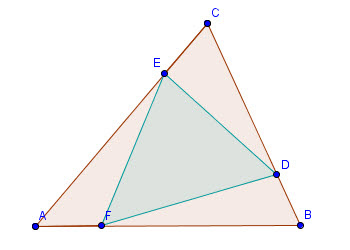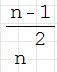## Sunday, 9 January 2011

### The "Nedial" TriangleI wrote recently about the concept of a Nedian; an extension of the idea of medians created by Professor John Satterly of the University of Toronto. I have played around a little more with the concept and come up with a calculation for the area of what I call a nedial triangle, extending the idea of a medial triangle.

Most High School students are introduced to the Medians of a triangle, and it is quite easy to show using basic high school geometry that the triangle whose vertices are the three feet of the medians has an area equal to 1/4 the area of the original triangle. In fact, it is pretty easy to show that the original triangle can be dissected into four congruent copies of the medial triangle.If instead we use the feet of the three nedians, we get a triangle I have called the nedial triangle. I have worked out that the area of such a triangle for an n-nedian (the cevian that cuts the opposite side 1/n th of the way along the edge) will have an area oftimes the area of the original triangle ABC. Since the median is the 2-Nedian, this gives the correct ratio for the medial triangles.

The image below shows the 4-nedial triangle, which has an area of 7/16 the area of ABC.The area of the three triangles at the vertices of the original triangle will each betimes the area of ABC.

The area of the nedial triangle will grow from a minimum of 1/4 the area of ABC increasing toward a limit of one as n approaches infinity. This can be confirmed by L'Hopital's rule or simple division of the terms. The fact that the limit is also one as n approaches one points out that when the base of the nedian is more than half way along the opposite side and the "n" in the nedian must be less than 2 but more than one we are measuring the area of what Satterly called the "backward nedians" which will create a nedial triangle of the same area. n=3/2 gives the exact area of n=3 (although the triangles are NOT congruent). In general for every k-nedial the area given by the nedial with index equal to n/n-1 has the same area.